The GENMOD Procedure

ESTIMATE Statement

• ESTIMATE 'label' contrast-specification </ options> ;

The ESTIMATE statement is similar to a CONTRAST statement, except only one-row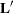matrices are permitted.

The elements of the ESTIMATE statement are as follows:

label

identifies the contrast on the output. A label is required for every contrast specified. Labels can be up to 20 characters and must be enclosed in single quotes.

contrast-specification

identifies the effects and their coefficients from which thematrix is formed. The contrast-specification can be specified in two different ways. The first method applies to all models except the zero-inflated (ZI) distributions (zero-inflated Poisson and zero-inflated negative binomial), and the syntax is:

effect values <…effect values>

The second method of specifying a contrast applies only to ZI models, and the syntax is:

effect values <…effect values> @ZERO effect values <…effect values>

where

effect

identifies an effect that appears in the MODEL statement. The value INTERCEPT or intercept can be used as an effect when an intercept is included in the model. You do not need to include all effects that are included in the MODEL statement.

values

are constants that are elements of thevector associated with the effect.

options

specifies options for the ESTIMATE statement.

For ZI models, sets of effects values before the @ZERO separator correspond to the regression part of the model with regression parameters, and effects values after the @ZERO separator correspond to the zero-inflation part of the model with regression parameters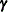. In the case of ZI models, a one-rowmatrix is created for the regression part of the model, another one-rowmatrix is created for the zero-inflation part of the model, and separate estimates for the twomatrices are computed and displayed.

If you use the default less-than-full-rank GLM CLASS variable parameterization, each row is checked for estimability. If PROC GENMOD finds a contrast to be nonestimable, it displays missing values in corresponding rows in the results. See Searle (1971) for a discussion of estimable functions.

The actual estimate,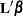(and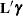for ZI models), its approximate standard error, and confidence limits are displayed. Additionally, the corresponding estimate on the mean scale (defined as the inverse link function applied to), and confidence limits are displayed. Wald chi-square tests that= 0 and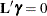are also displayed.

The approximate standard error of the estimate is computed as the square root of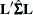, where
is the estimated covariance matrix of the parameter estimates. If you specify a GEE model in the REPEATED statement,is the empirical covariance matrix estimate.

If you specify the EXP option, then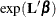, its standard error, and its confidence limits are also displayed.

The construction of thevector and the checking for estimability for an ESTIMATE statement follow the same rules as listed under the CONTRAST statement.

You can specify the following options in the ESTIMATE statement after a slash (/).

ALPHA=number

requests that a confidence interval be constructed with confidence level 1 – number. The value of number must be between 0 and 1; the default value is 0.05.

DIVISOR=number

specifies a value by which to divide all coefficients so that fractional coefficients can be entered as integer numerators. For example, you can use

estimate '1/3(A1+A2) - 2/3A3' a 1 1 -2 / divisor=3;


estimate '1/3(A1+A2) - 2/3A3' a 0.33333 0.33333 -0.66667;

E

requests that thematrix coefficients be displayed.

EXP

requests that, its standard error, and its confidence limits be computed. If you specify the EXP option, standard errors are computed using the delta method. Confidence limits are computed by exponentiating the confidence limits for.

SINGULAR=number
EPSILON=number

tunes the estimability checking as described for the CONTRAST statement.